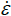Table T1. Nomenclature.

Variable Definition Dimension Unit
Cc Compression index Dimensionless
Cs Swelling index Dimensionless
cv Coefficient of consolidation L2/T m2/s
D Particle diameter L m
D50 Median particle diameter L mm
e Void ratio Dimensionless
e0 Reference void ratio Dimensionless
eσa Void ratio at axial effective stress Dimensionless
g Acceleration due to gravity L/T2 m/s2
H Instantaneous sample height L m
H0 Initial sample height L m
k Permeability L2 m2
k0 Permeability at reference void ratio L2 m2
L Effective depth from solution surface to center of hydrometer bulb L m
mp Mass fraction of particles remaining in suspension Dimensionless
mv Coefficient of volume compressibility LT2/M 1/Pa
Pc Consolidation cell pressure M/LT2 Pa
Pp Pore pressure M/LT2 Pa
t Time T s
V Volume of solution L3 m3
Δu Base excess pressure M/LT2 Pa
ε Axial strain Dimensionless
εσa Axial strain at axial effective stress DimensionlessStrain rate 1/T 1/s
µ Viscosity M/LT Pa·s